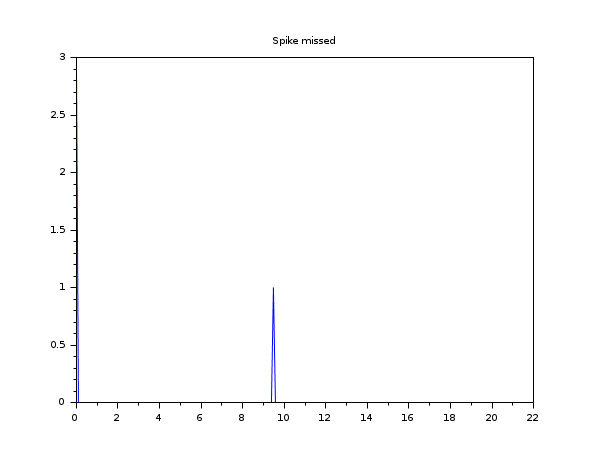Scilab Home page | Wiki | Bug tracker | Forge | Mailing list archives | ATOMS | File exchange
Please login or create an account
Change language to: Français - Português - 日本語 - Русский

Please note that the recommended version of Scilab is 6.1.0. This page might be outdated.
See the recommended documentation of this function

# intg

definite integral

### Syntax

`[v, err [,ierr]]=intg(a, b, f [,ea [,er]])`

### Arguments

a, b

real numbers.

f

external (function or list or string).

ea, er

real numbers.

ea

absolute error required on the result. Default value: 1.d-14.

er

relative error required on the result. Default value: 1.d-8.

err

estimated absolute error on the result.

ierr

error flag number (= 0 if no error occurred).

### Description

`intg(a,b,f)` evaluates the definite integral from `a` to `b` of `f(t)dt`. The function `f(t)` should be continuous.

The evaluation hopefully satisfies following claim for accuracy: `abs(I-v)<= max(ea,er*abs(I))` where `I` stands for the exact value of the integral.

`f` is an external :

If `f` is function its definition must be as follows `y = f(t)`

If `f` is a list the list must be as follows: `list(f,x1,x2,...)` where `f` is a function with syntax `f(t,x1,x2,...)`.

If `f` is a string it refers to the name of a Fortran function or a C procedure with a given syntax:

In the fortran case the calling sequence should be ```double precision function f(x)``` where `x` is also a double precision number.

In the C case the syntax should be ```double f(double *x)``` .

### Known Limitation

Like all the integrators, `intg` is subject to spike missing.

A flat function with a spike will be seen as a fully flat function if the spike is stiff enough.

This cannot be bypassed, it is easy to understand why when we know how the integrator operates. Indeed, `intg` uses the 21-point Gauss-Kronrod rule, so if there is a spike in-between two consecutive integration points, then it will go undetected, the function will be considered smooth.

However, a warning message will be issued if the function is considered very smooth. The user will then be suggested to reduce the integration interval, should he think that spikes were missed.

The following graphs illustrate that phenomenon.Being in-between the 9th and 10th integration points, that spike is not detected and `intg` considers the function flat. In the next image, the spike is large enough to be covered by the integration points.If the user wants to display the computed solution even if the solver has encountered an error, he should add the third output argument `ierr`, that will transform the errors into warnings. This is mostly used in the case of rounding errors.

### Examples

```// Function written in the Scilab language
function y=f(x),y=x*sin(30*x)/sqrt(1-((x/(2*%pi))^2)),endfunction
exact=-2.5432596188;
I=intg(0,2*%pi,f)
abs(exact-I)

// Function with an argument written in the Scilab language
function y=f1(x, w),y=x*sin(w*x)/sqrt(1-((x/(2*%pi))^2)),endfunction
I=intg(0,2*%pi,list(f1,30))
abs(exact-I)

// Function written in Fortran (a Fortran compiler is required)
// define a Fortran function
cd TMPDIR;
F=['      double precision function ffun(x)'
'      double precision x,pi'
'      pi=3.14159265358979312d+0'
'      ffun=x*sin(30.0d+0*x)/sqrt(1.0d+0-(x/(2.0d+0*pi))**2)'
'      return'
'      end'];
mputl(F,fullfile(TMPDIR,'ffun.f'))

// compile the function
l=ilib_for_link('ffun',fullfile(TMPDIR,'ffun.f'),[],'f');

// add the function to the working environment
link(l,'ffun','f')

// integrate the function
I=intg(0,2*%pi,'ffun')
abs(exact-I)

// Function written in C (a C compiler is required)
// define a C function
C=['#include <math.h>'
'double cfun(double *x)'
'{'
'  double y,pi=3.14159265358979312;'
'  y=*x/(2.0e0*pi);'
'  return *x*sin(30.0e0**x)/sqrt(1.0e0-y*y);'
'}'];
mputl(C,fullfile(TMPDIR,'cfun.c'))

// compile the function
l=ilib_for_link('cfun',fullfile(TMPDIR,'cfun.c'),[],'c');

// add the function to the working environment
link(l,'cfun','c')

// integrate the function
I=intg(0,2*%pi,'cfun')
abs(exact-I)```

### See also

• intc — Cauchy integral
• intl — Cauchy integral
• inttrap — integration of experimental data by trapezoidal interpolation
• intsplin — integration of experimental data by spline interpolation
• ode — ordinary differential equation solver

### Used Functions

The associated routines can be found in SCI/modules/differential_equations/src/fortran directory :

dqags.f and dqagse.f from quadpack

### Comments

Add a comment:
Please login to comment this page.

 Report an issue << integrate Differential calculus, Integration intl >>

 Scilab EnterprisesCopyright (c) 2011-2017 (Scilab Enterprises)Copyright (c) 1989-2012 (INRIA)Copyright (c) 1989-2007 (ENPC)with contributors Last updated:Tue Feb 14 15:02:42 CET 2017